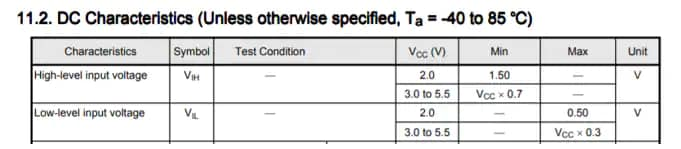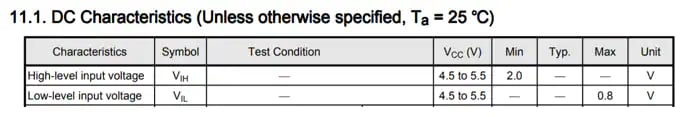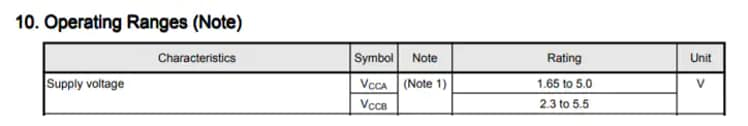# What options do I have to perform level shifting from 5 V to 3.3 V and vice versa?

The following figure shows level shifting from 5 V to 3.3 V and from 3.3 V to 5 V.

Down translation (High-to-low voltage level translation):
The input-tolerant function allows level shifting from a higher voltage to a lower voltage such as from 5 V to 3.3 V. For example, the input-tolerant function allows 5 V to be applied to an input without drawing extra current even when VCC = 0 V.Example of input specifications of a typical CMOS logic IC with a non-TTL input (74VHC240)

For down translation using the input-tolerant function of a CMOS logic IC, ensure that the maximum low-level input voltage is within the VIL range.Up translation using a CMOS logic IC with a TTL input

Up translation (Low-to-High voltage level translation):
Level shifting from a lower voltage to a higher voltage such as from 3.3 V to 5 V can be performed if the high-level output voltage (VOH) of the driving IC satisfies the high-level input voltage (VIH) of the driven IC because VOH is almost equal to the supply voltage (VCC).
Since the minimum high-level input voltage (VIH) of typical CMOS logic ICs is specified as VCC × 0.7, VIH is 3.5 V when VCC = 5 V. Therefore, if the VOH of the driving IC is 3.3 V, the minimum VIH of the driven IC is not satisfied. In this case, consider using a CMOS logic IC with a TTL input.Example of TTL input specifications of a CMOS logic IC (74VHCT240)

The following shows the specifications of the TTL input of the 74HCT240. Since its minimum high-level input voltage (VIH) is 2.0 V, the 74HCT240 allows up translation from 3.3 V to 5 VUp translation from 2.0 V to 5.0 V using a level shifter

The 74VHCT240 cannot be used if the supply voltage of the preceding IC is lower than 2 V. In that case, it is necessary to use a level shifter.Example of a supply voltage range of a level shifter (TC7MPB9326)

There is also a method of Up translation using a product with open drain output. Due to the pull-up resistor Rpull, the output swings to the VCC level.

A new window will open